## I have a problem about integration

### Quick description

Clicking on the answers to questions below will take you to text and further questions.

Which of the following descriptions best fits the problem you are trying to solve?

I have an indefinite integral and I want to evaluate it. In that case, you have some function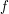and you would like to find a function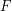that differentiates to. There is no systematic way of doing this, but there are plenty of tricks, such as integration by substitution and integration by parts. For plenty of integration tricks, try the Tricki page on evaluating indefinite integrals.

I have a definite integral and I want to evaluate it.

• Are you integrating over an interval of finite length or an interval of infinite length?
• Of finite length.
• For an integral of the form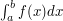, probably your best bet is to evaluate the corresponding indefinite integral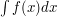, using the advice about evaluating indefinite integrals, to find a functionthat differentiates to, in which case the answer is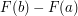. Another technique that sometimes works is differentiation under the integral sign: you differentiate the integral with respect to some variable, obtain a different quantity that is easier to integrate, and integrate it to get your answer. And another is to use an appropriate series expansion: you write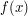as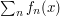, integrate the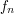, and add up the results.
• Of infinite length
• For an integral such as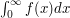, the best method may be to find a functionthat differentiates toand to take the answer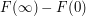, assuming that you can make good sense of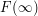by an appropriate limiting argument. However, you have other options as well, such as contour integration and differentiation under the integral sign.

I have a definite integral that looks impossible to evaluate exactly in terms of standard functions, but I want to approximate it.

I have an integralover an interval of infinite length and I want to show that the integral is finite.

• Which of the following statements seems more likely in your case?
• The integral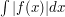is finite as well.
• In other words, you believe that your function is absolutely integrable. In that case, the single most important technique (apart from direct evaluation if that turns out to be possible) is the continuous analogue of the comparison test for infinite sums: you find a non-negative function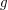such that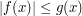for everyand such that you can prove that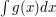is finite. There are many ways of implementing this basic idea: see bound the integrand by something simpler for details.
• The integralis not finite, butoscillates enough for the resulting cancellation to causeto be finite.
• In that case, you have what is known as an oscillatory integral. An example of such an integral is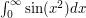, which is finite because it oscillates faster and faster, while remaining bounded, so the function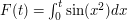makes smaller and smaller oscillations and converges to a limit. See methods for bounding oscillatory integrals for details about how to handle this kind of situation.

I have an integralover an interval of finite length and I want to show that it is finite.

• Which of the following statements best describes your problem?
• The functionis bounded.
• In that case, you should be able to bound your integral by its base times its height. If you can't, then pretty well the only thing that can have gone wrong is that your function isn't integrable in the first place. See the later advice on proving that a function is integrable.
• The integralis not finite, but a positive blow-up on one side of a point is sort of compensated for by a negative blow-up on the other side.
• An example of such an integral is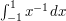. Strictly speaking, this is neither Riemann integrable (because it is unbounded) nor Lebesgue integrable (because it is not absolutely integrable). However, the limit of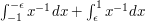exists (and is equal to). This is an improper integral. When it is less obvious that the limit exists, you may need to use tools for estimating integrals. An example would be the function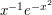, say, which is also improperly integrable between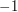and. Improper integrals are important in Fourier analysis, as they arise in the definition of the Hilbert transform.

I need to prove that a functionis Riemann integrable.

• Which of the following statements best describes the problem?
• The function is given by an explicit formula.
• Is the function continuous?
• Yes.
• Which of the following statements best describes the function?
• It is defined on a closed bounded interval.
• then all you need to do is use the theorem that a continuous function defined on a closed bounded interval is Riemann integrable.
• It is defined on an interval that is bounded but not closed.
• If you can extend it continuously to a closed interval, then you can use the theorem that a continuous function defined on a closed bounded interval is Riemann integrable. If your function is bounded, but cannot be extended continuously to the endpoint, then it is still integrable. To find out how to prove this, then look at the discussion of functions about which one just has certain assumptions. If your function is unbounded, then it is not Riemann integrable, but it may be that a limit such as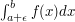exists. To determine this, you will need to think about methods for estimating integrals.
• It is defined on a closed but unbounded interval.
• One would normally say that the function is Riemann integrable if the interval of integration can be split up into intervals of finite length on which your function is integrable. By this definition, your function is Riemann integrable. If you are worried about the integral possibly being infinite, then you should look at earlier advice about proving that an integral is finite.
• No.
• Which of the following statements best describes the function?
• It is continuous except at finitely many points.
• Assuming it is bounded (and if it is not bounded then it is not Riemann integrable), then if what you have is jump discontinuities, then all you need to do is look at finitely many subintervals separately, in each one defining your function at the end points in a way that makes it continuous. If you have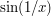-type discontinuities, then your best bet is to forget the precise definition of the function and prove the general result that a bounded function that is continuous except at finitely many points is Riemann integrable. This kind of proof is discussed below.
• It has infinitely many discontinuities.
• I am not actually told what the function is, but just given some assumptions about it.
• The advice to give here depends very much on what you are allowed to assume. For instance, often it is a good idea to use the theorem that a continuous function on a closed bounded interval is Riemann integrable. But if that is precisely the result you want to know how to prove, then obviously you can't use it. So let us get a feel for what exactly your problem is.
• I have just been given the definition of the Riemann integral and want to prove from first principles thatis Riemann integrable.
• Almost always, the best approach is to use Riemann's criterion: a functiondefined on the interval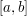is Riemann integrable if and only if the following statement holds. For every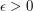there exists a dissection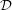such that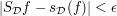, where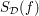is the upper sum associated withand the dissection, and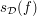is the lower sum. For more details, see how to prove from first principles that a function is Riemann integrable.
• I am happy to assume a few basic facts about Riemann integrable functions.
• Three basic facts, or collections of facts, you might use are these. First, a continuous function defined on a closed bounded interval is Riemann integrable. Secondly, a bounded monotone function defined on a bounded interval is Riemann integrable. Thirdly, ifandare integrable on, then you can make other integrable functions such as linear combinations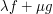, the pointwise product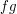, and so on.

If these results are not enough to solve your problem, then there is a very useful principle that may be. It's that bounded functions that behave almost everywhere are Riemann integrable. This, for example, can be used to show that the functiondefined on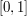that equalswhen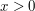andwhen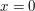is Riemann integrable: this function is bounded, and it is continuous everywhere except at.

I have a functionthat I am told is Riemann integrable, and I want to prove something about.

• At the time of writing, I cannot think of any useful general advice for dealing with this situation, except perhaps to use Riemann's criterion. But here is a very nice problem to think about: let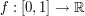be a Riemann integrable function and suppose that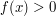for every. Prove that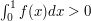.